AP Calculus AB : Asymptotic and Unbounded Behavior

Example Questions

Example Question #20 : Asymptotic Behavior In Terms Of Limits Involving Infinity

Find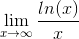.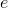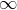Explanation:

First step for finding limits: evaluate the function at the limit.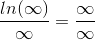which is an Indeterminate Form.

This got us nowhere. However, since we have an indeterminate form, we can use L'Hopital's Rule (take the derivative of the top and bottom and the limit's value won't change).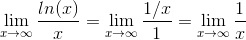This is something we can evaluate: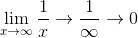The value of this limit is.

Example Question #21 : Asymptotic And Unbounded Behavior

Assume that a population of bunnies grows at a rate of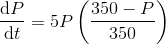whereis the number of bunnies in the population at time. If the population begins with 14 bunnies, given unlimited time to grow, how many bunnies do you expect there to be in the population?

Note: No integration is required for this problem.

70

700The population will never stop growing.

5

350

350

Explanation:

The equation given is a model of logistic growth. Note that one of the other common forms this equation might be given in is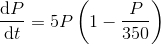. What we want to know is what the population will be given unlimited time, or what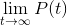is.

Looking at the form of the derivative above, note that if we start with 14 bunnies, we get a positive derivative. This means that the population is increasing. As our function is continuous, the population will keep growing until the derivative hits 0. When does this occur?

In the above form, it should be more clear that the derivative is only 0 at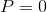and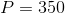. Thus, the population will keep growing, but never go above 350, because if it were to hit 350, the derivative would be 0 and growth would stop. (Alternatively, if the population were above 350, you can see the derivative would be negative and that the population would shrink back down to 350. Indeed, it wasn't necessary to tell you we started with 14 bunnies -- the limit will be the same for any positive starting value.)

Example Question #22 : Asymptotic Behavior In Terms Of Limits Involving Infinity

The function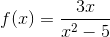has a horizontal asymptote at y=0. What does the presence of the horizontal asymptote imply?

At x=0 the function is undefined.

The function is discontinuous at y=0.

The limit of the function as x-values approach 0 tends to either positive or negative infinity.

The function will produce only positive outputs.

Explanation:

Unlike vertical asymptotes, horizontal asymptotes of certain functions may be crossed. In these cases, and in the case of the given function, the horizontal asymptote may be crossed and even have defined values laying on it (e.g., f(0)=0 for the function provided).

What is meaningful about the horizontal asymptote in this example is that it suggests the behavior of the function at large magnitudes. The denominator will increase much faster than the numerator. That is to say that the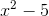will grow exponentially larger thein the numerator such that, at large values (both positive and negative), the function will output y-values tending toward y=0.

Example Question #1 : Comparing Relative Magnitudes Of Functions And Their Rates Of Change

Evaluate the definite integral of the algebraic function.

integral (x+ √(x))dx from 0 to 1

11/12

1

5/12

0

10/12

11/12

Explanation:

Step 1: Rewrite the problem.

integral (x3+x1/2) dx from 0 to 1

Step 2: Integrate

x4/4 + 2x(2/3)/3 from 0 to 1

Step 3: Plug in bounds and solve.

[14/4 + 2(1)(2/3)/3] – [04/4 + 2(0)(2/3)/3] = (1/4) + (2/3) = (3/12) + (8/12) = 11/12

Example Question #1 : Comparing Relative Magnitudes Of Functions And Their Rates Of Change

Evaluate the integral.

Integral from 1 to 2 of (1/x3) dx

–3/8

0

1/2

–5/8

3/8

3/8

Explanation:

Integral from 1 to 2 of (1/x3) dx

Integral from 1 to 2 of (x-3) dx

Integrate the integral.

from 1 to 2 of (x–2/-2)

(2–2/–2) – (1–2/–2) = (–1/8) – (–1/2)=(3/8)

Example Question #1 : Comparing Relative Magnitudes Of Functions And Their Rates Of Change

Evaluate the following indefinite integral.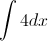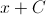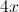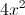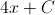Explanation:

In order to evaluate the indefinite integral, ask yourself, "what expression do I differentiate to get 4".  Next, use the power rule and increase the power ofby 1. To start, we have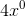, so in the answer we have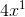.  Next add a constant that would be lost in the differentiation.  To check your work, differentiate your answer and see that it matches "4".

Example Question #4 : Comparing Relative Magnitudes Of Functions And Their Rates Of Change

Evaluate the following indefinite integral.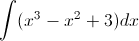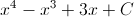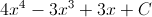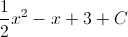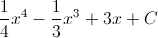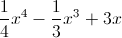Explanation:

Use the inverse Power Rule to evaluate the integral.  We know that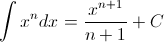for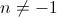.  We see that this rule tells us to increase the power ofby 1 and multiply by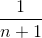.  Next always add your constant of integration that would be lost in the differentiation.  Take the derivative of your answer to check your work.

Example Question #1 : Comparing Relative Magnitudes Of Functions And Their Rates Of Change

Evaluate the following indefinite integral.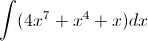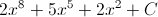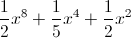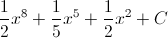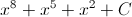Explanation:

Use the inverse Power Rule to evaluate the integral.  We know thatfor.  We see that this rule tells us to increase the power ofby 1 and multiply by.  Next always add your constant of integration that would be lost in the differentiation.  Take the derivative of your answer to check your work.

Example Question #21 : Asymptotic And Unbounded Behavior

Evaluate the following indefinite integral.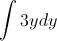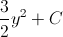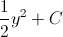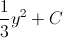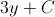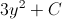Explanation:

Use the inverse Power Rule to evaluate the integral.  Firstly, constants can be taken out of integrals, so we pull the 3 out front.  Next, according to the inverse power rule, we know thatfor.  We see that this rule tells us to increase the power ofby 1 and multiply by. Next always add your constant of integration that would be lost in the differentiation.  Take the derivative of your answer to check your work.

Example Question #2 : Comparing Relative Magnitudes Of Functions And Their Rates Of Change

Evaluate the following indefinite integral.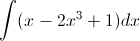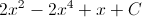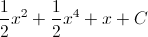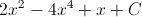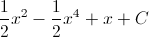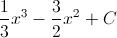Use the inverse Power Rule to evaluate the integral.  We know thatfor.  We see that this rule tells us to increase the power ofby 1 and multiply by.  Next always add your constant of integration that would be lost in the differentiation.  Take the derivative of your answer to check your work.